# Spontaneity, Entropy, and Free EnergyPage 2

#### WATCH ALL SLIDES

G0 = H0 - TS0

Calculating Free Energy Method #1

Slide 10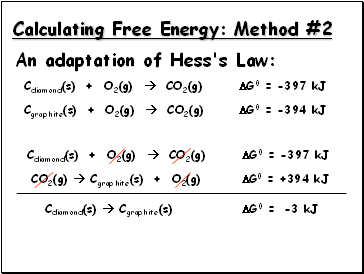Calculating Free Energy: Method #2

Cdiamond(s) + O2(g)  CO2(g) G0 = -397 kJ

Cgraphite(s) + O2(g)  CO2(g) G0 = -394 kJ

CO2(g)  Cgraphite(s) + O2(g) G0 = +394 kJ

Cdiamond(s)  Cgraphite(s)

G0 =

Cdiamond(s) + O2(g)  CO2(g) G0 = -397 kJ

-3 kJ

Slide 11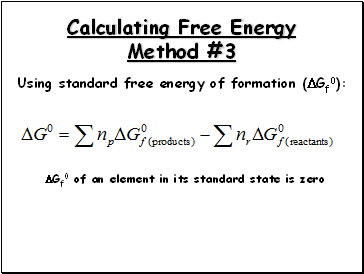Calculating Free Energy Method #3

Using standard free energy of formation (Gf0):

Gf0 of an element in its standard state is zero

Slide 12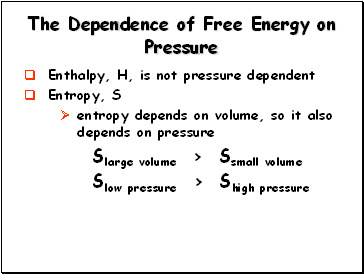## The Dependence of Free Energy on Pressure

Enthalpy, H, is not pressure dependent

Entropy, S

entropy depends on volume, so it also depends on pressure

Slarge volume > Ssmall volume

Slow pressure > Shigh pressure

Slide 13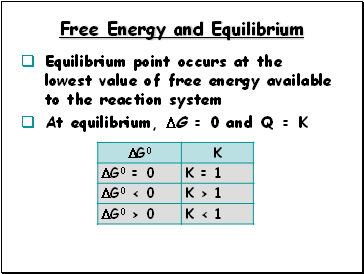## Free Energy and Equilibrium

Equilibrium point occurs at the lowest value of free energy available to the reaction system

At equilibrium, G = 0 and Q = K

Slide 14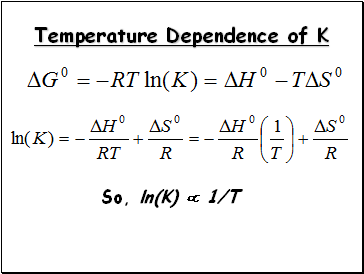## Temperature Dependence of K

So, ln(K)  1/T

Slide 15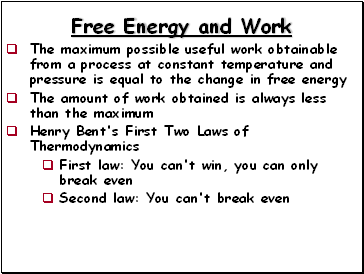## Free Energy and Work

The maximum possible useful work obtainable from a process at constant temperature and pressure is equal to the change in free energy

The amount of work obtained is always less than the maximum

Henry Bent's First Two Laws of Thermodynamics

First law: You can't win, you can only break even

Second law: You can't break even

Go to page:
1  2